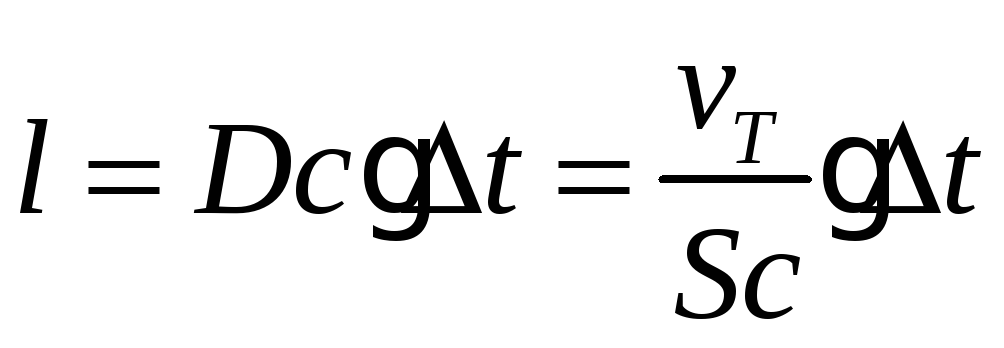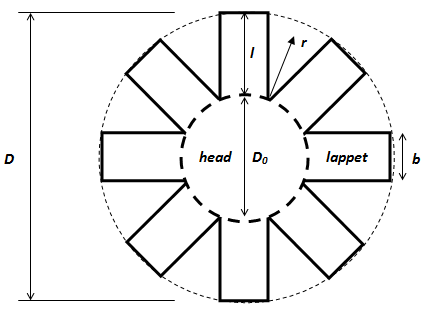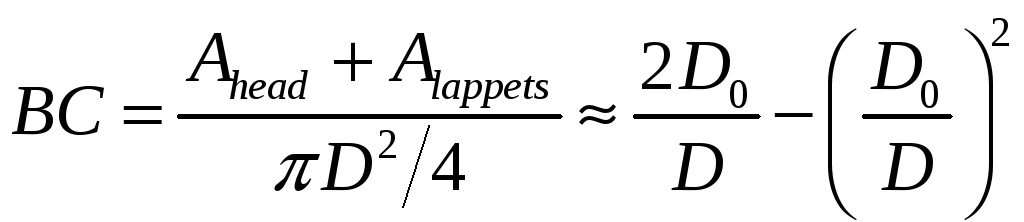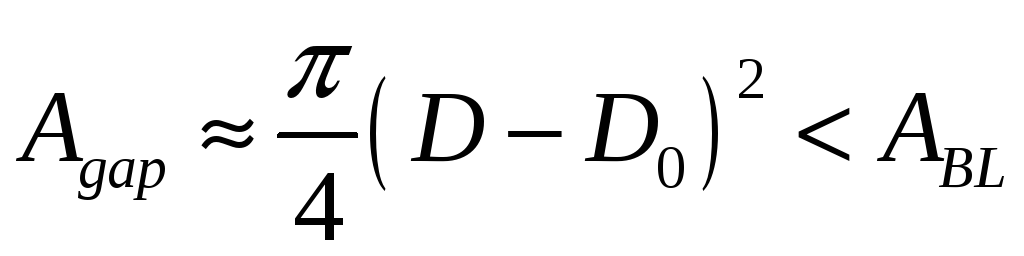# Supplemental Materials Dye visualization of boundary layer

 Supplemental Materials Dye visualization of boundary layer We assume that fluorescein dye is transported at the local water velocity (see error discussion below). Therefore, the local dye concentration will change according to the flow velocity profile and, in particular, highlight regions where the flow velocity differs from the free stream. This is the case for the boundary layers surrounding the jellyfish lappets. Here, the water velocity is similar to the lappet velocity such that the dye remains for a long time in the vicinity of the lappet whereas the water outside the boundary layer moves at velocities different from the lappet (i.e. at free stream velocities) allowing the dye to be advected away. The dye that appears to stick to the lappets therefore outlines the extent of the fluid layer moving at similar velocities as the lappet, i.e. the boundary layer (Koehl et al. 2001). The error of the boundary layer visualization can be estimated by the distance the dye will diffuse during the duration of one pulse and bell relaxation (and thereby blur fluid boundaries between strokes). Diffusion distance l during time period Δt can be derived bywhere Dc and Sc are the diffusion constant and Schmidt number, respectively. With Sc=2000 for fluorescein (Lavertu et al. 2008), we find at T=21°C with  ν21=1.05 ·10−6m2s−1 and pulse & relaxation time Δt=0.7 seconds, that the dye diffuses over a distance of l=4·10−10m. This is 5 orders of magnitude less than the theoretical boundary layer thickness of ca. 4·10−5m for the dimensions and morphology of the G13 that we assayed (calculated from equation (4) in the main paper with b=0.5mm, r=1mm and C=0.8). Similar relations are found for 13°C. Therefore, dye transport is dominated by advection. We conclude that the visualization error due to diffusion is negligible compared to boundary layer thicknesses as well as compared to the differences seen in overlap at different temperatures (figure 2a,b in main paper). Stokes model (additional details) We simplify the body plan of an ephyrae to a shape made of an inner circle, or “head”, and eight rectangular lappets (Feitl et al. 2009):The bell continuity BC, i.e. the percentage of the circular bell that is filled in with tissue is(1) Fluid dynamic constraint: In order for the medusa body to function as a continuous surface, the boundary layers between the lappets must fill the gaps, i.e.,(2) The boundary layer area Share with your friends: